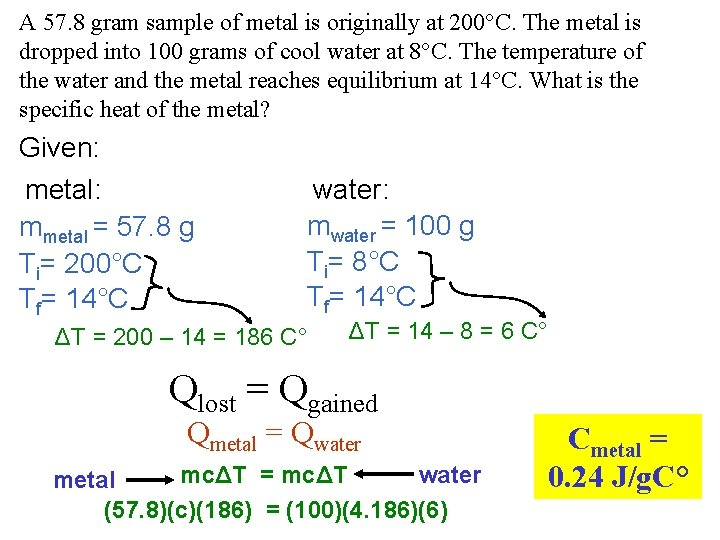# Temperature Scales Fahrenheit Celsius Kelvin 32 F freezing

• Slides: 8Temperature Scales Fahrenheit Celsius Kelvin - 32 F = freezing point of water 212 F = boiling point of water 0 C = freezing point of water 100 C = boiling point of water 273 K = freezing point of water 373 K = boiling point of water Absolute zero The temperature at which it is no longer possible to extract heat from the atoms Absolute zero = 0 K = - 273 C = - 460 FSpecific Heat Capacity Specific heat capacity is a measure of the ability of a material to absorb heat energy - specific heat capacity is defined as the amount of heat energy required to change the temperature of 1 gram of a substance by 1 C° Units of Specific Heat Capacity joules / gram·C° or calories / gram·C° Example: The specific heat capacity of water is 1 calorie / gram·C° This means it takes 1 calorie of heat energy to change the temperature of 1 gram of water by 1 C°Q = mcΔT Where: Q = heat m = mass c = specific heat capacity ΔT = temperature changeLatent Heat the heat energy involved in a phase change fusion (hf) – phase change from solid to liquid vaporization (hv) – phase change from liquid to gas Units of Latent Heat joules / gram For H 2 O: or calories / gram cwater= 4. 18 J/gram·Cº cice = 2. 01 J/gram·Cº csteam = 1. 97 J/gram·Cº hf = 334 J/gram hv = 2260 J/gramThe Heating Curve A → B increase in temperature of the solid (Q=mciceΔT) B → C melting of solid (Q=mhf) C → D increase in temperature of the liquid (Q=mcwaterΔT) D → E vaporization of liquid (Q=mhv) E → F increase in temperature of the vapor (Q=mcsteamΔT)How much heat is required to change a 6 gram ice cube at – 11°C to water at 44 °C? Q = mciceΔT + mhf + mcwaterΔT raise ice to 0°C melt ice raise water (melted ice) to 44°C Q = (6)(2. 01)(11) + 6(334) + 6(4. 186)(44) = 3242 J THE LAW OF HEAT EXCHANGE Qlost = Qgained When you use the law of heat exchange, the change in temperature (ΔT) must be positive.A 57. 8 gram sample of metal is originally at 200°C. The metal is dropped into 100 grams of cool water at 8°C. The temperature of the water and the metal reaches equilibrium at 14°C. What is the specific heat of the metal? Given: metal: mmetal = 57. 8 g Ti= 200°C Tf= 14°C water: mwater = 100 g Ti= 8°C Tf= 14°C ΔT = 200 – 14 = 186 C° ΔT = 14 – 8 = 6 C° Qlost = Qgained Qmetal = Qwater mcΔT = mcΔT water metal (57. 8)(c)(186) = (100)(4. 186)(6) Cmetal = 0. 24 J/g. C°A 50 gram piece of brass (c= 0. 092 cal/g. C°) at 95°C is dropped into 250 grams of water at 17. 0°C in an 85 gram glass (c= 0. 20 cal/g. C°) beaker. What is the final temperature of everything? Given: metal: water: mmetal = 50. 0 g cmetal= 0. 092 cal/g. C° Ti= 95°C mwater = 250 g cwater = 1. 00 cal/g. C° Ti= 17°C beaker: mbeaker = 85 g cbeaker = 0. 20 cal/g. C° Ti= 17°C 50(0. 092)(95 - Tf) = 250(1. 00)(Tf - 17) + 85(0. 20)(Tf - 17) Qlost = Qgained 4. 6(95 - Tf) = 250(Tf - 17) + 17(Tf - 17) Qmetal = Qwater + Qbeaker DISTRIBUTE mcΔT = mcΔT + mcΔT 437 - 4. 6 Tf = 250 Tf - 4250 + 17 Tf - 289 4976 = 271. 6 Tf Tf = 18. 3°C metal water beaker